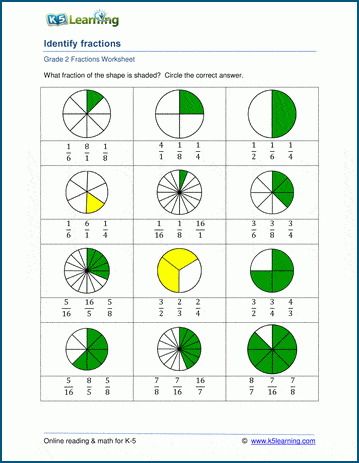# Identifying fractions with pictures

## Matching fractions worksheets: parts of a whole

These grade 2 math worksheets give students practice in matching written fractions to divided shapes.  Fractions are most easily understood as "parts of a whole". In these exercises, students are given simple shapes split into equal parts and must circle the correct fraction as written in standard form.## More fractions worksheets

Explore all of our fractions worksheets, from dividing shapes into "equal parts" to multiplying and dividing improper fractions and mixed numbers.

## What is K5?

K5 Learning offers reading and math worksheets, workbooks and an online reading and math program for kids in kindergarten to grade 5.  We help your children build good study habits and excel in school.